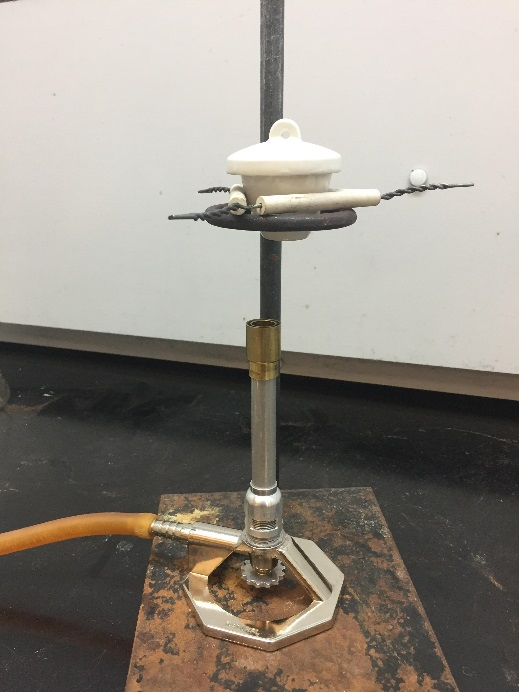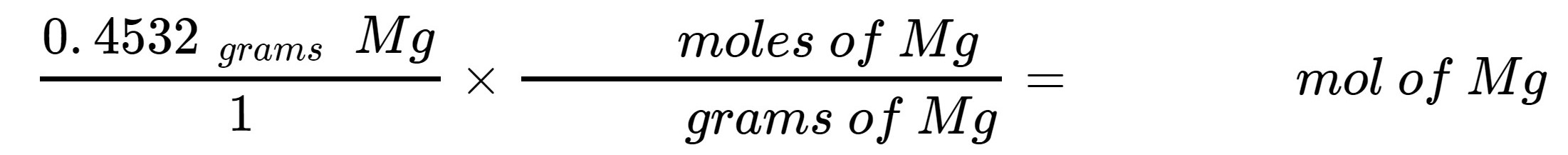# Experiment_602_Empirical Formula of MgO 1_4_2

$$\newcommand{\vecs}{\overset { \rightharpoonup} {\mathbf{#1}} }$$ $$\newcommand{\vecd}{\overset{-\!-\!\rightharpoonup}{\vphantom{a}\smash {#1}}}$$$$\newcommand{\id}{\mathrm{id}}$$ $$\newcommand{\Span}{\mathrm{span}}$$ $$\newcommand{\kernel}{\mathrm{null}\,}$$ $$\newcommand{\range}{\mathrm{range}\,}$$ $$\newcommand{\RealPart}{\mathrm{Re}}$$ $$\newcommand{\ImaginaryPart}{\mathrm{Im}}$$ $$\newcommand{\Argument}{\mathrm{Arg}}$$ $$\newcommand{\norm}{\| #1 \|}$$ $$\newcommand{\inner}{\langle #1, #2 \rangle}$$ $$\newcommand{\Span}{\mathrm{span}}$$ $$\newcommand{\id}{\mathrm{id}}$$ $$\newcommand{\Span}{\mathrm{span}}$$ $$\newcommand{\kernel}{\mathrm{null}\,}$$ $$\newcommand{\range}{\mathrm{range}\,}$$ $$\newcommand{\RealPart}{\mathrm{Re}}$$ $$\newcommand{\ImaginaryPart}{\mathrm{Im}}$$ $$\newcommand{\Argument}{\mathrm{Arg}}$$ $$\newcommand{\norm}{\| #1 \|}$$ $$\newcommand{\inner}{\langle #1, #2 \rangle}$$ $$\newcommand{\Span}{\mathrm{span}}$$$$\newcommand{\AA}{\unicode[.8,0]{x212B}}$$

 Student Name Laboratory Date:  Date Report Submitted: ___________________________ Student ID Experiment Number and Title Experiment 602:  Empirical Formula

Experiment 602:  Empirical Formula

Section 1:  Purpose and Summary

• Determine the empirical formula of magnesium oxide.

• Calculate the mass of oxygen using weighing-by-difference.

• Calculate the mole of a sample from its mass.

In this experiment, students will conduct the reaction between magnesium and oxygen gas.  Students will determine the mass of magnesium sample before and after the reaction, and the mass of magnesium and oxygen in the product.  Students will learn how to convert mass to mole of a given sample and determine empirical formula of a substance from mass and mole data.

Section 2:  Safety Precautions and Waste Disposal

Safety Precautions:

Do not look directly at the burning magnesium ribbon. The flame is bright enough to damage your eye.  Use of eye protection is required for all experimental procedures.

A hot crucible will break if placed directly on a cold surface.  Set hot crucibles on to wire screens to cool.

A hot crucible will break if splashed with water directly.   Let crucibles cool prior to adding water.

Waste Disposal:

The solid product from the reaction can be disposed into the regular garbage can in the lab.

Section 3: Procedure

Part 1:  Preparation of the crucible

 Heat a clean and dry porcelain crucible with lid (on a clay triangle supported on a ring stand) directly over a Bunsen burner flame for about 5 minutes. This will ensure that the crucible is clean and dry.     Here is what the setup should look like:Turn off the burner and allow the crucible to cool. Leave the crucible resting on the clay triangle. DO NOT put a hot crucible on the lab bench. When the crucible is cool to touch, weigh the crucible and lid on the digital balance.     It is important to use a balance that measures to 0.0001 grams.  If you use the wrong balance, you will have to repeat this experiment. Mass of empty crucible and lid:    (a)________________ grams

Part 2 Preparation of magnesium sample

 Obtain a strip or two of magnesium ribbon, about 6.0 to 7.0 cm long.     NOTE: If it is not shiny, polish it slightly with steel wool to remove any oxide coating.      Use sufficient magnesium strips to mass between 0.4 and 0.6 grams on an analytical balance (a balance that measures to 0.0001 g) Fold or loosely coil the magnesium ribbon(s) to make sure it sits entirely on the bottom of the crucible. Put the coiled magnesium ribbon into the crucible, cover, and weigh on the digital balance. Record mass. Mass of crucible, magnesium ribbon, and lid:    (b)________________ grams

Part 3:  Heating the magnesium sample

 Place the crucible containing the magnesium ribbon back into the clay triangle. Put the lid close by on the lab bench, with crucible tongs to handle it with later. Light the Bunsen burner and start heating the crucible and magnesium ribbon. As soon as the magnesium ribbon starts to burn, put the lid back (using crucible tongs) to put out the flame. Continue heating the crucible with the lid on for a minute or so, then remove the lid again. When the magnesium ribbon starts to burn again, put the lid back to put out the flame. Repeat this heating process until the magnesium ribbon no longer catches fire. With the lid on, heat the crucible and sample strongly for 5 minutes. Make sure that the bottom of the crucible turns ‘red’ hot. Turn off the burner, and let the crucible, lid, and sample cool on the clay triangle. When the crucible is cool to touch, remove the lid, then add about 10 drops of laboratory water into the burned magnesium ribbon. Make sure to wet the entire sample, not just one spot. Light the Bunsen burner and heat the crucible (without lid) with a low flame for a minute or so.   This evaporates the water you just added.  After the water has evaporated, increase the flame and heat it strongly for about 10 minutes. The bottom of the crucible does not need to turn ‘red’ hot during this heating. Turn off the burner, and the let the crucible and the sample cool on the clay triangle. When the crucible is cool to touch, weigh the crucible, product, and lid on the digital balance. Mass of crucible, product, and lid:     (c)________________ grams

Section 4:  Calculations

 From the masses you recorded in Parts 1 and 2 of this experiment, calculate the mass of magnesium ribbon.  (b) – (a) (d) _________________  g Mg From the masses you recorded in Parts 2 and 3 of this experiment, calculate the mass of magnesium oxide produced from the reaction.  (c) – (a) (e) _________________ g MgO From the mass of magnesium oxide (e) and the mass of magnesium ribbon (d), calculate the mass of oxygen that combined with magnesium during the reaction.  (e) – (d) (f) ________________ g O Refer to a periodic table to obtain the molar mass of magnesium. Then, using this information and the mass of magnesium ribbon (d), calculate the number of moles of magnesium that you started with.      Molar mass of Mg: ___________g/mol (from a Periodic Table)    Show your equation here:            EXAMPLE:  If a student used 0.4532 g of Mg, then set up your equation like this.  Use your molar mass of Mg value.   Set up the equation so that the units cancel.(g) ____________  mol Mg Obtain the molar mass of oxygen from a periodic table.  Using this information and the mass of oxygen you calculated (f), calculate the number of moles of oxygen that combined with the magnesium.    Molar mass of O: ____________ g/mol (from a periodic table)    Show your equation here:          EXAMPLE:  If a student measured a mass gain of 0.3005 g due to the oxygen combusted, then set up your equation like this.  Use your molar mass of oxygen value.   Set up the equation so that the units cancel.      $$\frac{\text {0.3005 grams} \quad \text {O}}{1} \times \frac{\text {moles of O}}{\text {grams of O}}=$$ mol of O (h) _______________  mol O Calculate the mole ratio between magnesium and oxygen by dividing the mol Mg (g) by the mol O (h).     Show your equation here:          EXAMPLE:  If a student measured x moles of Mg and y moles of O, the equation should be set up as Set up the equation so that the units cancel.      $$\frac{\text {x} \quad \text {moles of Mg}}{\text {y} \quad \text {moles of O}}=$$ mole ratio of Mg to O in magnesium oxide (i) ________________ Using your mole ratio determined in (i), write the empirical formula of magnesium oxide. Round off the ratio to the nearest whole number. Empirical Formula:

Post Lab Questions:

1.  There are some experimental errors that could lead to high or low mole ratio between Mg and O. In each case below, decide whether the situation described would lead to a calculated ratio of too much oxygen, or too little oxygen, and explain your answer.

(a) You forgot to do the initial drying step and proceeded right away to weighing the crucible and lid you obtained from the stockroom.

(b) Your magnesium ribbon is not shiny. But you did not polish it with steel wool prior to use as indicated in the experiment.

(c) You added more laboratory water than is needed in Part 3 Step 7, and you did not dry it out completely.

(d) After strong heating of the crucible you removed the lid but dropped it and broke. You then obtained a new lid for the final weighing.

2. A similar experiment is performed to determine the empirical formula of an oxide of copper, and the following data were collected. Predict the empirical formula of the copper oxide from these data.

Mass of crucible, cover, and copper sample 21.53 g

Mass of empty crucible with cover 19.66 g

Mass of crucible and cover and sample (after heating) 21.76 g

How can the experiment for the determination of the empirical formula of an oxide of copper be improved?

Experiment_602_Empirical Formula of MgO 1_4_2 is shared under a CC BY license and was authored, remixed, and/or curated by LibreTexts.